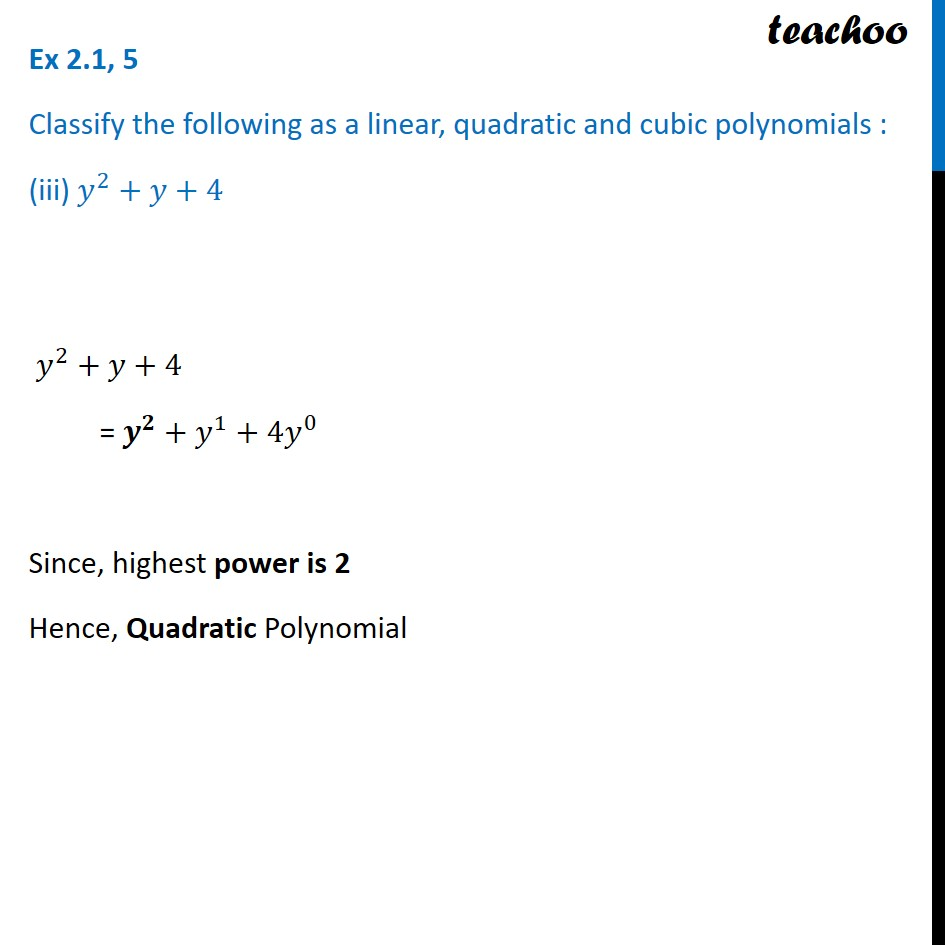Ex 2.1

Chapter 2 Class 9 Polynomials
Serial order wiseLearn in your speed, with individual attention - Teachoo Maths 1-on-1 Class

### Transcript

Ex 2.1, 5 Classify the following as a linear, quadratic and cubic polynomials : (iii) 𝑦^2+𝑦+4 𝑦^2+𝑦+4 = 𝒚^𝟐+𝑦^1+4𝑦^0 Since, highest power is 2 Hence, Quadratic Polynomial